Like   Tweet   Pin   +1   in

## Elder E-Letter: Winter 2019

 table.module-2{width:80%;padding:0}table div table+table+table div table{width:80%;float:none;margin-left:auto;margin-right:auto;padding:0}table div table+table+table div table a{border:0 none;text-decoration:none}table div table+table+table div table img{width:100%!important;border:0 none;text-decoration:none}table div table+table+table div table td{width:100%;padding:0}/* styles */

## Happy New Year from The Law Offices of Carol Bertsch, PC!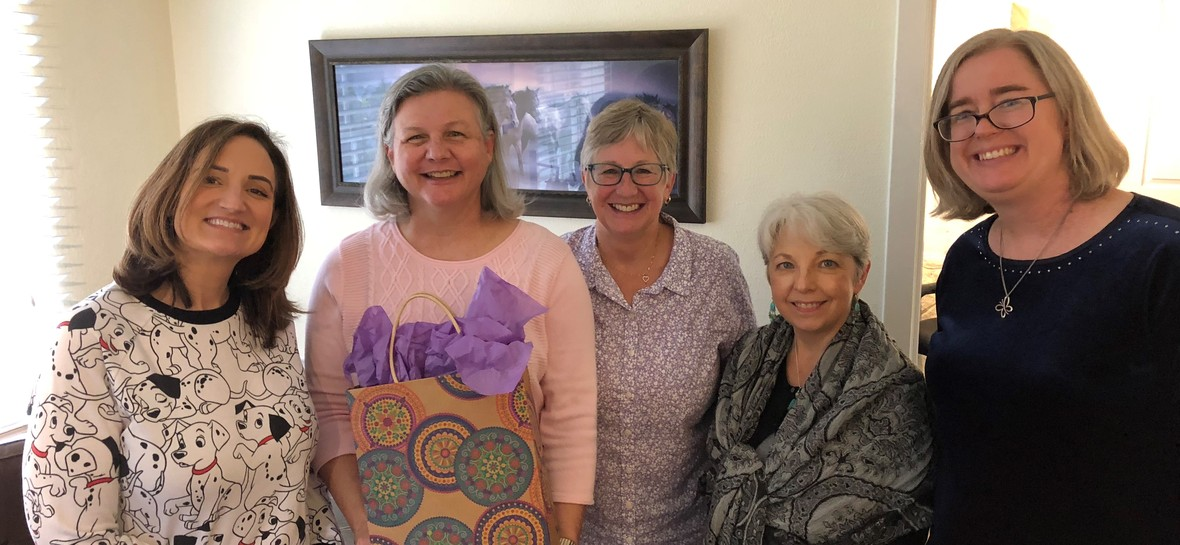table div table+table+table+table+table+table div table{width:100%;padding:0}table div table+table+table+table+table+table div table img{width:96.23%;padding:0;float:none}table div table+table+table+table+table+table div table td{width:100%;padding:0 1.88% 18px}/* styles */## Save the Date: The Alzheimer's Association Annual Educational Summit on Alzheimer's and Related Dementias

 /* styles */ When: Friday, March 29, 2019 7:30 AM to 4:00 PM Family Caregivers, Professionals, and the General Public are invited to attend the Alzheimer's Association Annual Educational Summit. For those of you who came last year, you already know this is going to be an awesome event. Registration includes: educational materials, food, CEU's for professionals, and access to experts in the field. This event is free for caregivers. Register here Location: Oblate School of Theology Whitley Center 285 Oblate Drive San Antonio, TX 78216
 table div table+table+table+table+table+table+table+table+table div table{width:100%;padding:0}table div table+table+table+table+table+table+table+table+table div table img{width:96.23%;padding:0;float:none}table div table+table+table+table+table+table+table+table+table div table td{width:100%;padding:0 1.88% 18px}/* styles */## Paralegal Toni O'Brien Elected Treasurer of First Friday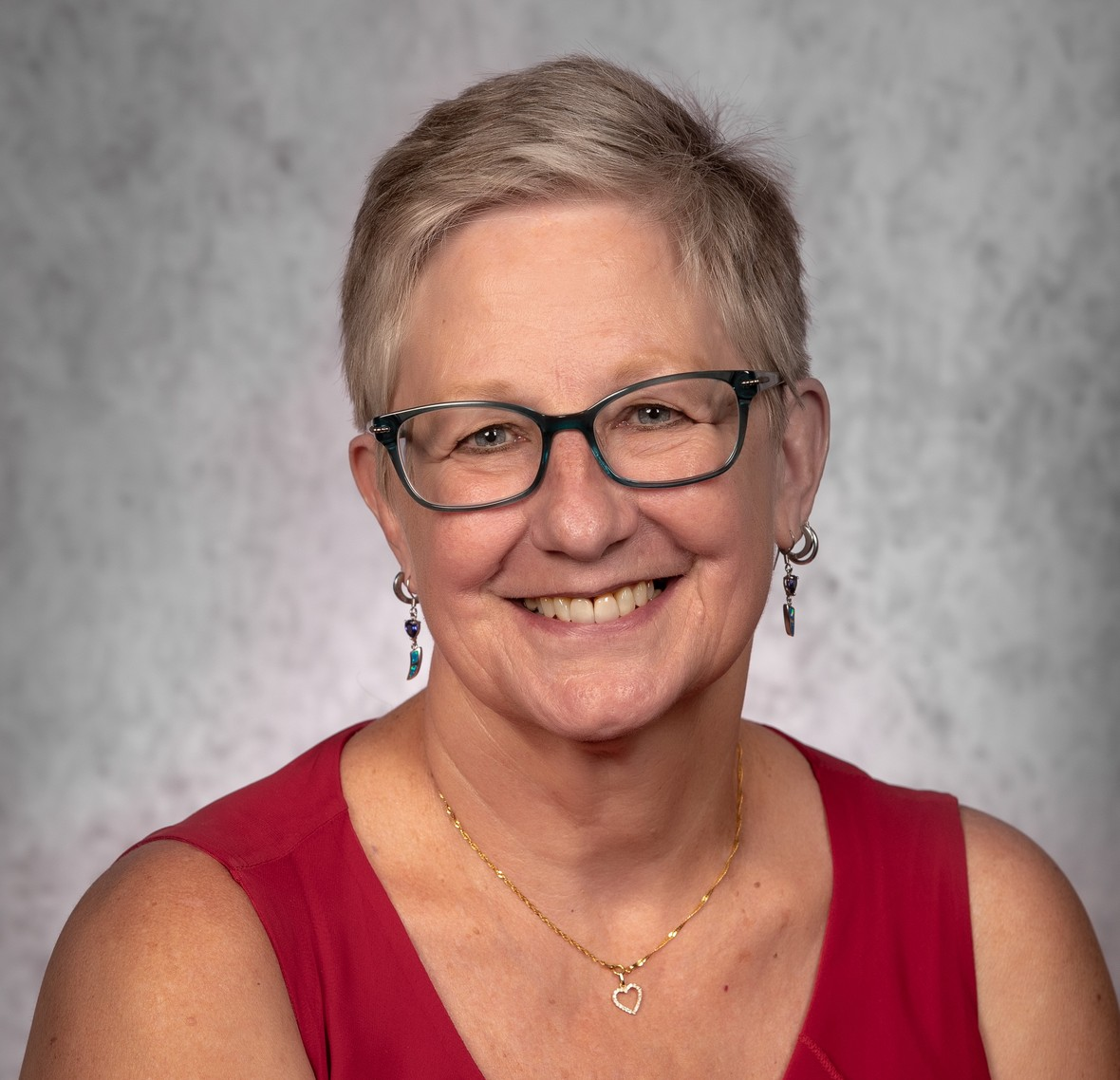/* styles */ Toni O'Brien was elected as First Friday Treasurer. First Friday is a business networking group for senior care professionals in San Antonio and the surrounding areas.
 table div table+table+table+table+table+table+table+table+table+table+table+table+table div table{width:100%;padding:0}table div table+table+table+table+table+table+table+table+table+table+table+table+table div table img{width:96.23%;padding:0;float:none}table div table+table+table+table+table+table+table+table+table+table+table+table+table div table td{width:100%;padding:0 1.88% 18px}/* styles */table div table+table+table+table+table+table+table+table+table+table+table+table+table+table+table+table+table div table{width:100%;padding:0}table div table+table+table+table+table+table+table+table+table+table+table+table+table+table+table+table+table div table img{width:96.23%;padding:0;float:none}table div table+table+table+table+table+table+table+table+table+table+table+table+table+table+table+table+table div table td{width:100%;padding:0 1.88% 18px}/* styles */## Coming up/* styles */ Jan. 1 - The Law Offices of Carol Bertsch, PC observes New Years Day Jan. 18-19 - Attorneys Ellen G. Patterson and Carol Bertsch attend the Texas NAELA UnProgram 2019/* styles */ Jan 19 - The most important day of the year—Carol’s birthday, of course! Happy Birthday, Carol!/* styles */ Jan 21 - The Law Offices of Carol Bertsch, PC observes Martin Luther King Jr. Day and will be walking with the NAACP at the annual Martin Luther King Jr. March Feb. 7-8 - Attorneys Ellen G. Patterson and Carol Bertsch attend the UT Law CLE on Special Needs Trusts Feb. 19 - Carol speaks at Oasis: Morningside about "Estate Planning: Wills & Trusts" (Register here by searching "Estate planning". Registration opens 1/04/19) Mar. 1 - Paralegal Toni O'Brien will be at the San Antonio Bar Association's Extreme Family Law Makeover representing The Law Offices of Carol Bertsch, PC Mar. 28 - Carol presents Special Needs Trusts to UT Health Science Center Mar. 29 - The Law Offices of Carol Bertsch, PC sponsors Alzheimer's Association Annual Education Summit. Come say hi to Paralegal Jody Hudspeth as she staffs the table!
 table div table+table+table+table+table+table+table+table+table+table+table+table+table+table+table+table+table+table+table+table+table+table+table+table+table div table{width:100%;padding:0}table div table+table+table+table+table+table+table+table+table+table+table+table+table+table+table+table+table+table+table+table+table+table+table+table+table div table img{width:96.23%;padding:0;float:none}table div table+table+table+table+table+table+table+table+table+table+table+table+table+table+table+table+table+table+table+table+table+table+table+table+table div table td{width:100%;padding:0 1.88% 18px}/* styles */## Happenings

 /* styles */ Nov. 1 - Carol spoke at the Caregiver SOS Summit on End of Life Planning Nov. 10 - The Law Offices of Carol Bertsch, PC supported the Run for Mom 5K for the Sarah Roberts French Home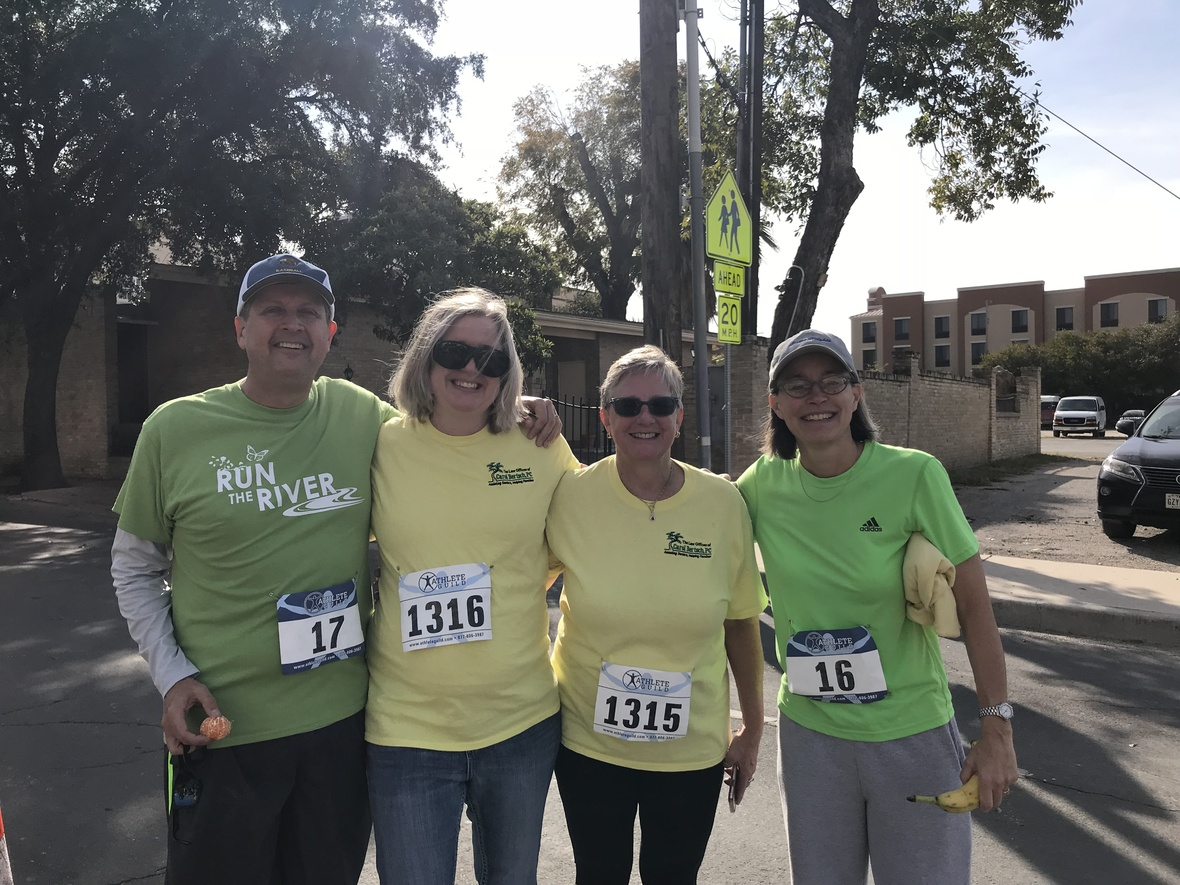/* styles */ Nov. 17 - Paralegal Toni O'Brien and Attorney Ellen Patterson ran the Green Spaces Alliance of South Texas Run the River 5K representing the Law Offices of Carol Bertsch, PC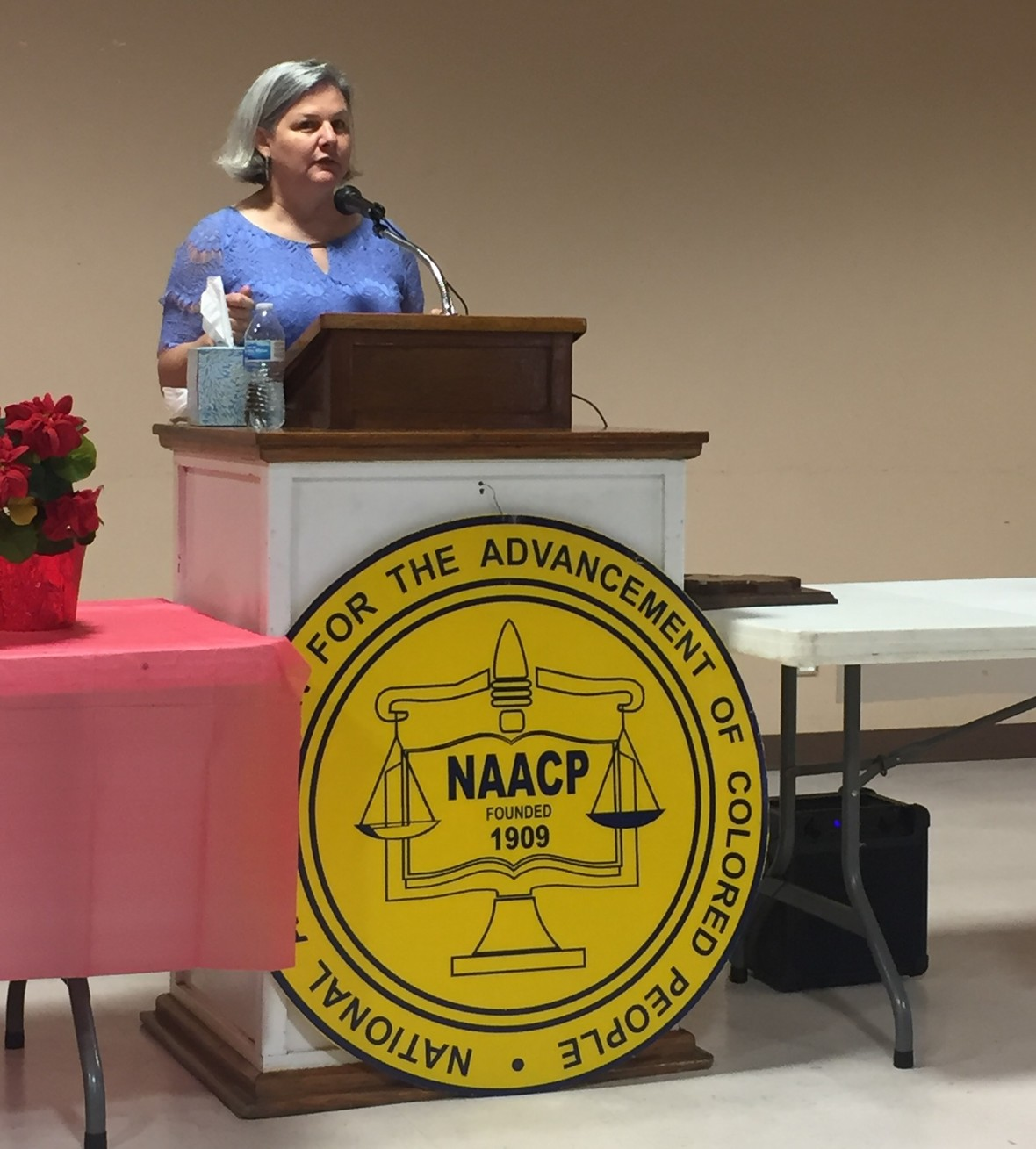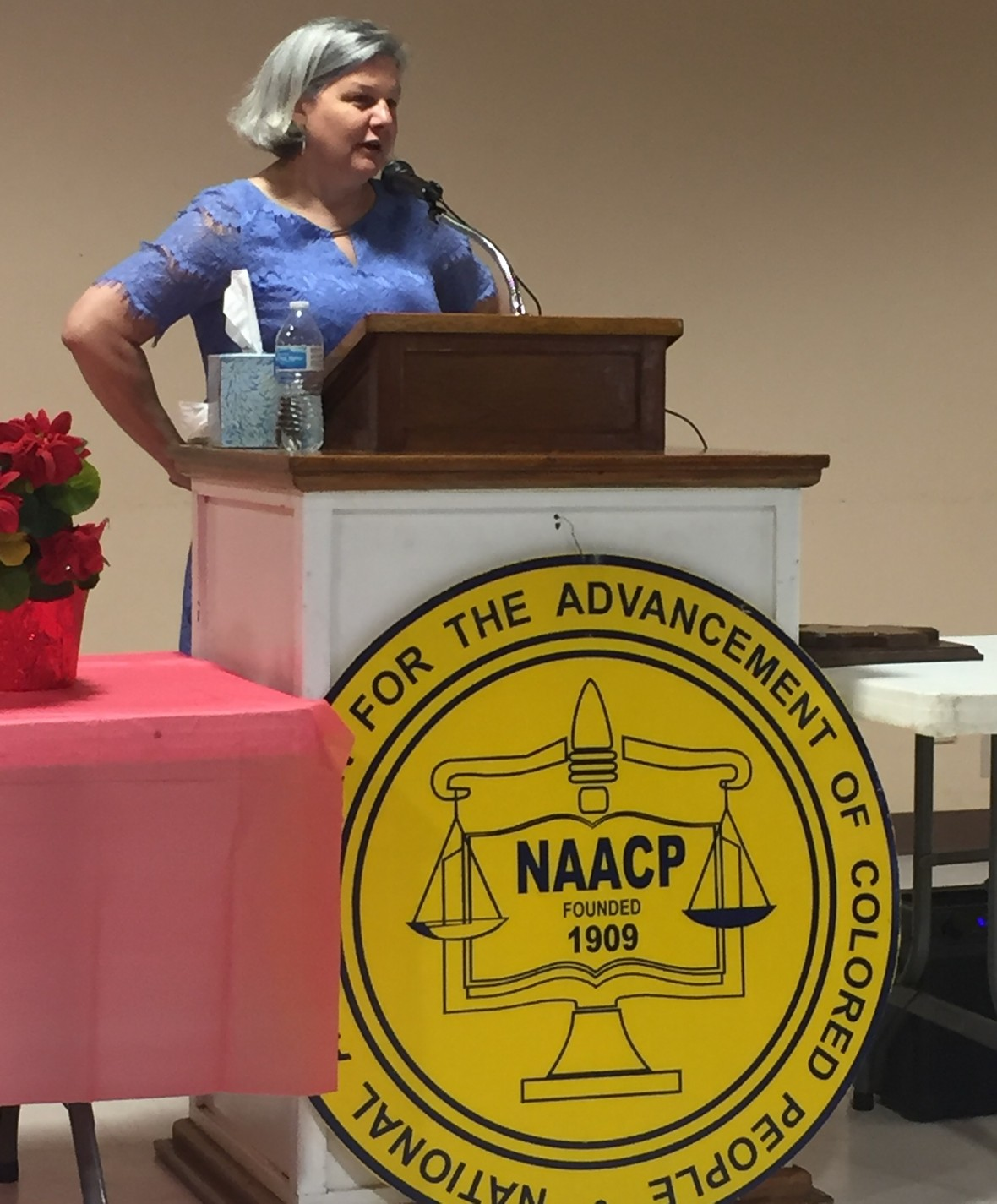/* styles */ Dec. 1 - Carol presented Getting Your Affairs in Order to seniors at the NAACP San Antonio Branch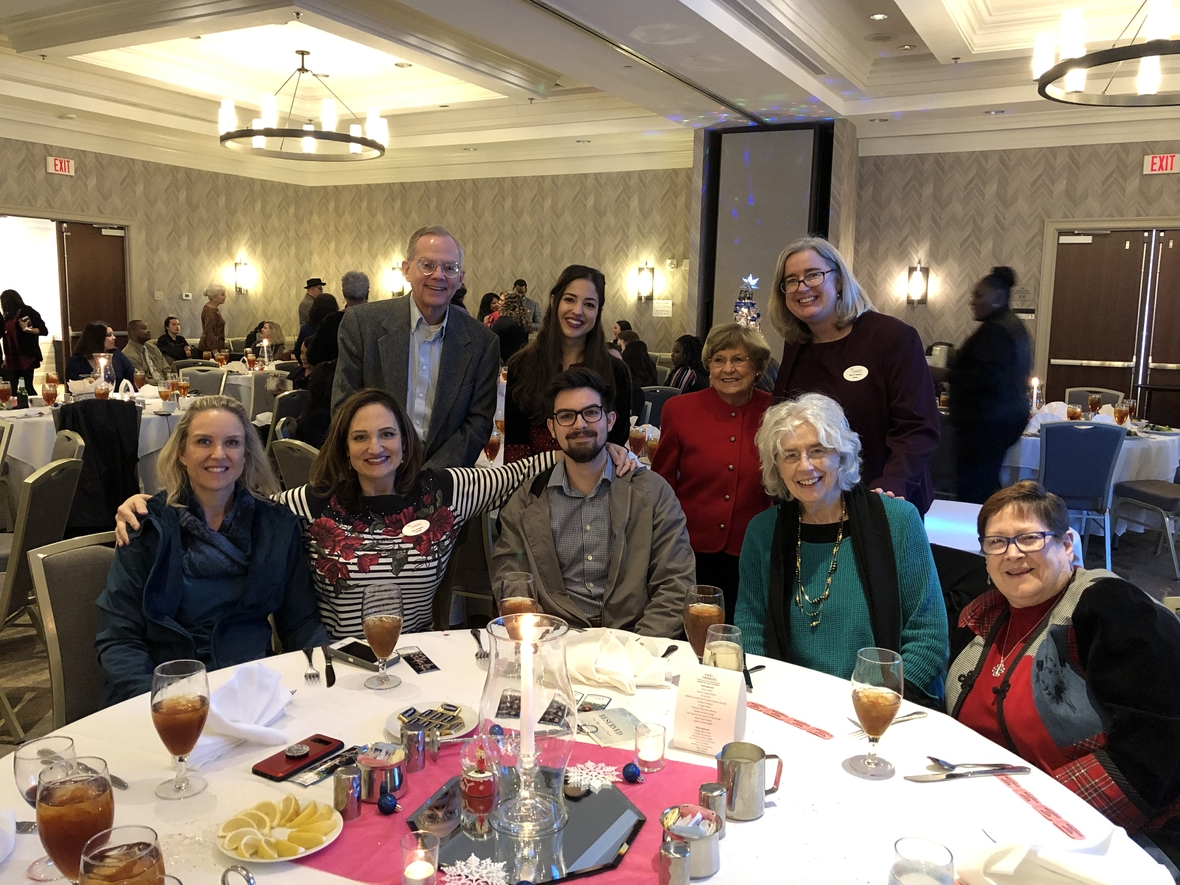/* styles */ Dec. 7 - The Law Offices of Carol Bertsch, PC was a Diamond sponsor for the AAHCC Winter Wonderland Christmas Party benefiting Jefferson Outreach
 table div table+table+table+table+table+table+table+table+table+table+table+table+table+table+table+table+table+table+table+table+table+table+table+table+table+table+table+table+table+table+table+table+table+table div table{width:100%;padding:0}table div table+table+table+table+table+table+table+table+table+table+table+table+table+table+table+table+table+table+table+table+table+table+table+table+table+table+table+table+table+table+table+table+table+table div table img{width:96.23%;padding:0;float:none}table div table+table+table+table+table+table+table+table+table+table+table+table+table+table+table+table+table+table+table+table+table+table+table+table+table+table+table+table+table+table+table+table+table+table div table td{width:100%;padding:0 1.88% 18px}/* styles */## Caregiver's Corner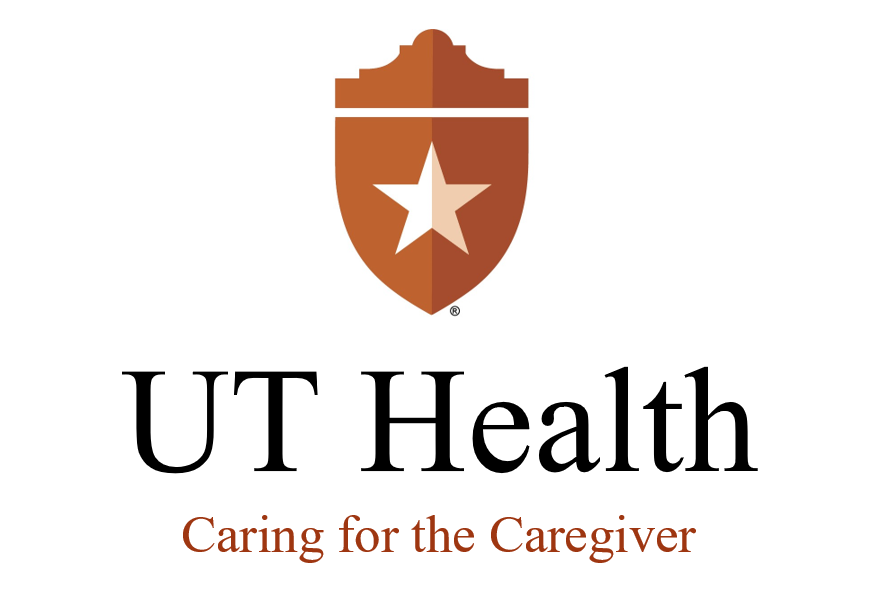## UT Health: Caring for the Caregiver

 /* styles */ Caring for the Caregiver is a great educational resource for caregivers. To view upcoming events visit the UT Caregivers Events Page.

## UT Health: Glenn Brigg's Institute

 /* styles */ UT Health's Glenn Brigg's Institute is another fantastic resource that hosts lectures about Alzheimer's. Visit the Brigg's Institute Events Page to view upcoming events.## Alzheimer's Association

 /* styles */ Click here for caregiver resources in Bexar County that are sponsored by the Alzheimer's Association.
 table div table+table+table+table+table+table+table+table+table+table+table+table+table+table+table+table+table+table+table+table+table+table+table+table+table+table+table+table+table+table+table+table+table+table+table+table+table+table+table+table+table+table+table+table div table{width:100%;padding:0}table div table+table+table+table+table+table+table+table+table+table+table+table+table+table+table+table+table+table+table+table+table+table+table+table+table+table+table+table+table+table+table+table+table+table+table+table+table+table+table+table+table+table+table+table div table img{width:96.23%;padding:0;float:none}table div table+table+table+table+table+table+table+table+table+table+table+table+table+table+table+table+table+table+table+table+table+table+table+table+table+table+table+table+table+table+table+table+table+table+table+table+table+table+table+table+table+table+table+table div table td{width:100%;padding:0 1.88% 18px}/* styles */## A Great Way to Combat Senior Isolation/* styles */ Senior Planet hosts technology and computer courses geared to people who are over 60. All of Senior Planet’s courses and events are free. Classes will begin in January 2019 that cover a range of topics, from health and wellness to social engagement. Please call (210)504-4862 for more information.
 table div table+table+table+table+table+table+table+table+table+table+table+table+table+table+table+table+table+table+table+table+table+table+table+table+table+table+table+table+table+table+table+table+table+table+table+table+table+table+table+table+table+table+table+table+table+table+table+table div table{width:100%;padding:0}table div table+table+table+table+table+table+table+table+table+table+table+table+table+table+table+table+table+table+table+table+table+table+table+table+table+table+table+table+table+table+table+table+table+table+table+table+table+table+table+table+table+table+table+table+table+table+table+table div table img{width:96.23%;padding:0;float:none}table div table+table+table+table+table+table+table+table+table+table+table+table+table+table+table+table+table+table+table+table+table+table+table+table+table+table+table+table+table+table+table+table+table+table+table+table+table+table+table+table+table+table+table+table+table+table+table+table div table td{width:100%;padding:0 1.88% 18px}/* styles */## Check Out This Helpful Medicare Resource/* styles */ Medicare Interactive is a clearly written and easy-to-understand website sponsored by the Medicare Rights Center. The website is a great information resource.
 table div table+table+table+table+table+table+table+table+table+table+table+table+table+table+table+table+table+table+table+table+table+table+table+table+table+table+table+table+table+table+table+table+table+table+table+table+table+table+table+table+table+table+table+table+table+table+table+table+table+table+table+table div table{width:100%;padding:0}table div table+table+table+table+table+table+table+table+table+table+table+table+table+table+table+table+table+table+table+table+table+table+table+table+table+table+table+table+table+table+table+table+table+table+table+table+table+table+table+table+table+table+table+table+table+table+table+table+table+table+table+table div table img{width:96.23%;padding:0;float:none}table div table+table+table+table+table+table+table+table+table+table+table+table+table+table+table+table+table+table+table+table+table+table+table+table+table+table+table+table+table+table+table+table+table+table+table+table+table+table+table+table+table+table+table+table+table+table+table+table+table+table+table+table div table td{width:100%;padding:0 1.88% 18px}/* styles */## What’s Going on in Your World?/* styles */ We appreciate your feedback. Do you have a book or a topic you'd like to see featured in our newsletter? Please email your suggestions to jhudspeth@assistingseniors.com. All ideas are welcome.
 table div table+table+table+table+table+table+table+table+table+table+table+table+table+table+table+table+table+table+table+table+table+table+table+table+table+table+table+table+table+table+table+table+table+table+table+table+table+table+table+table+table+table+table+table+table+table+table+table+table+table+table+table+table+table+table+table div table{width:100%;padding:0}table div table+table+table+table+table+table+table+table+table+table+table+table+table+table+table+table+table+table+table+table+table+table+table+table+table+table+table+table+table+table+table+table+table+table+table+table+table+table+table+table+table+table+table+table+table+table+table+table+table+table+table+table+table+table+table+table div table img{width:96.23%;padding:0;float:none}table div table+table+table+table+table+table+table+table+table+table+table+table+table+table+table+table+table+table+table+table+table+table+table+table+table+table+table+table+table+table+table+table+table+table+table+table+table+table+table+table+table+table+table+table+table+table+table+table+table+table+table+table+table+table+table+table div table td{width:100%;padding:0 1.88% 18px}/* styles */table div table+table+table+table+table+table+table+table+table+table+table+table+table+table+table+table+table+table+table+table+table+table+table+table+table+table+table+table+table+table+table+table+table+table+table+table+table+table+table+table+table+table+table+table+table+table+table+table+table+table+table+table+table+table+table+table+table div table,table.module-56{width:32.08%;float:left;padding:0}table div table+table+table+table+table+table+table+table+table+table+table+table+table+table+table+table+table+table+table+table+table+table+table+table+table+table+table+table+table+table+table+table+table+table+table+table+table+table+table+table+table+table+table+table+table+table+table+table+table+table+table+table+table+table+table+table+table div table a{border:0 none;text-decoration:none}table div table+table+table+table+table+table+table+table+table+table+table+table+table+table+table+table+table+table+table+table+table+table+table+table+table+table+table+table+table+table+table+table+table+table+table+table+table+table+table+table+table+table+table+table+table+table+table+table+table+table+table+table+table+table+table+table+table div table img{width:100%!important;border:0 none;text-decoration:none}table div table+table+table+table+table+table+table+table+table+table+table+table+table+table+table+table+table+table+table+table+table+table+table+table+table+table+table+table+table+table+table+table+table+table+table+table+table+table+table+table+table+table+table+table+table+table+table+table+table+table+table+table+table+table+table+table+table div table td{width:100%;padding:0 20px 20px 0}/* styles */